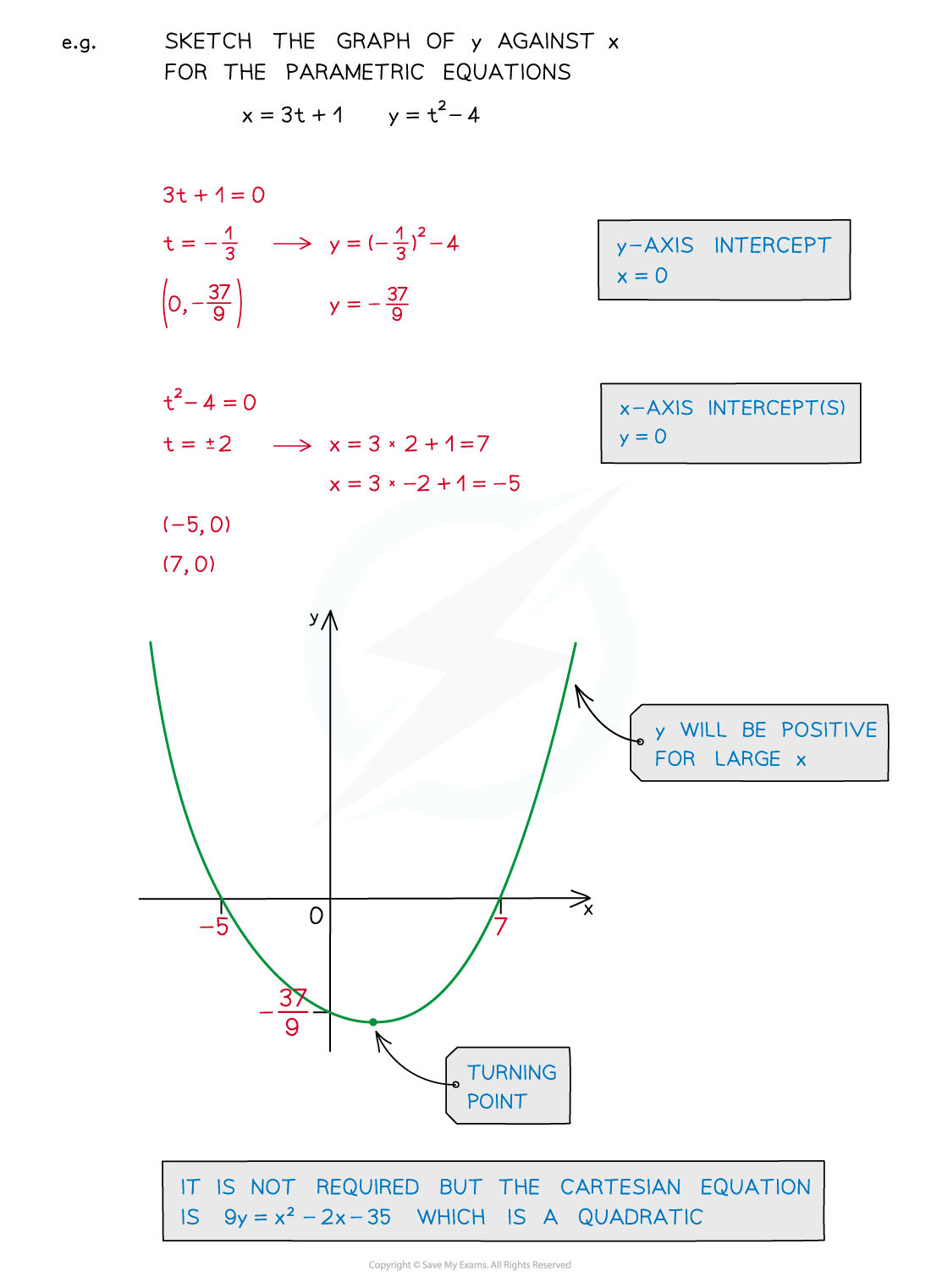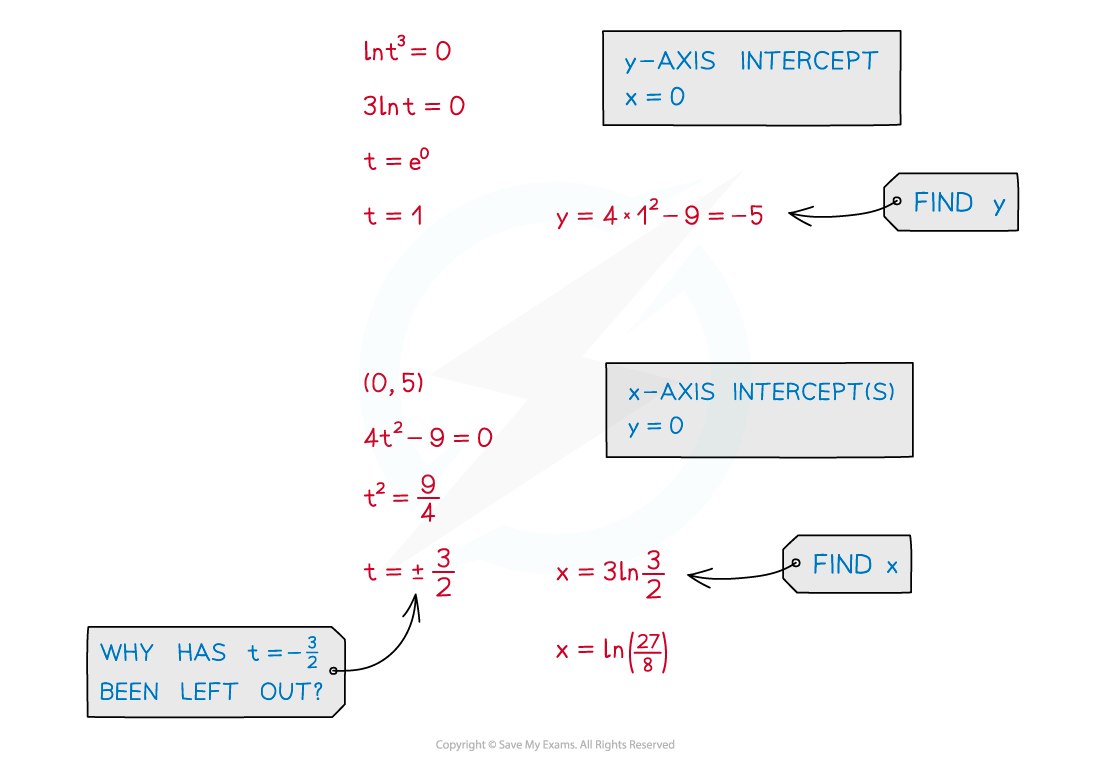# CIE A Level Maths: Pure 3复习笔记4.3.3 Parametric Equations - Sketching Graphs

### Parametric Equations - Sketching Graphs

#### How do I sketch a graph from parametric equations?

• Plotting a graph is covered in Parametric Equations - Basics• Still find the key features of a graph …
• … the y-axis intercept
• … the x-axis intercept(s)
• … asymptotes
• … location of (and if required coordinates of) stationary points (see Parametric Differentiation)
• Sketch these points and join up accordingly#### Exam Tip

• Not all curves defined parametrically lead to familiar shaped graphs and it may be worth plotting a few extra points by calculating them.
• Your calculator may be able to produce a table of values quickly, however, remember you are sketching and not plotting.
• It may be easier to find the Cartesian equation first and draw the graph from that – this will depend on the question.
• It is only a definite strategy if you cannot make progress otherwise.
• If you are given the sketch of a graph it is usually only for reference and can be used to check answers for axes intercepts, etc.

#### Worked Example